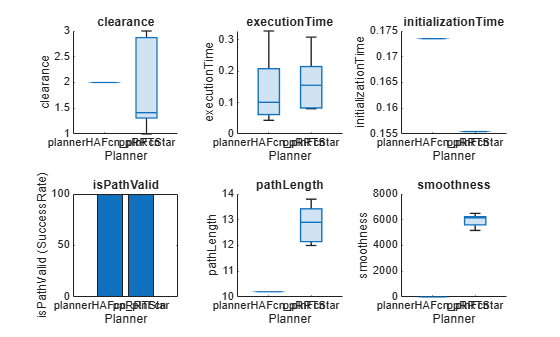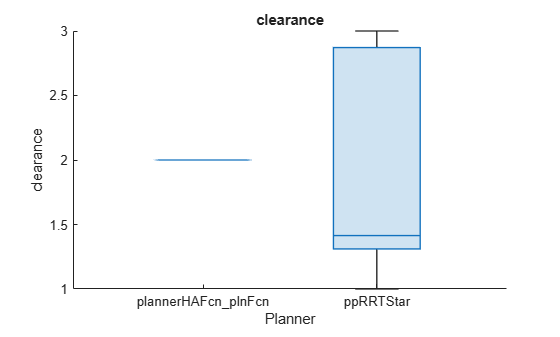# metric

Return path planner metrics

Since R2022a

## Syntax

``metricSummary = metric(plannerBM)``
``metricSummary = metric(plannerBM,metricName)``
``[metricSummary,metricData] = metric(plannerBM)``
``[metricSummary,metricData] = metric(plannerBM,metricName)``

## Description

````metricSummary = metric(plannerBM)` returns the summary of all the path planner metrics as a table.```
````metricSummary = metric(plannerBM,metricName)` returns the summary of a specific metric.```
````[metricSummary,metricData] = metric(plannerBM)` returns the `metricData` table with the metric values across each run for all metrics and `metricSummary` table with the summary of all metrics.```

example

````[metricSummary,metricData] = metric(plannerBM,metricName)` returns the summary and the values of a specific metric.```

## Examples

collapse all

Create an occupancy map from an example map.

```load("exampleMaps.mat","simpleMap"); map = occupancyMap(simpleMap);```

Create a state validator with `stateSpaceSE2` using the map.

`sv = validatorOccupancyMap(stateSpaceSE2,Map=map);`

Specify the start and goal states.

```start = [5 8 pi/2]; goal = [7 18 pi/2];```

Create a `plannerBenchmark` object.

`pbo = plannerBenchmark(sv,start,goal);`

Define the function handles for the initialization functions of the planners.

```plannerHAFcn = @(sv)plannerHybridAStar(sv); plannerRRTSFcn = @(sv)plannerRRTStar(sv.StateSpace,sv);```

Define the function handle for the plan function, which is common for both planners.

`plnFcn = @(initOut,s,g)plan(initOut,s,g);`

Add the path planners for benchmarking.

```addPlanner(pbo,plnFcn,plannerHAFcn); addPlanner(pbo,plnFcn,plannerRRTSFcn,PlannerName="ppRRTStar");```

Set the `rng` for repetitive results.

`rng('default')`

Run the path planners for the number of times specified in `runCount` to collect metrics.

```runCount = 5; runPlanner(pbo,runCount)```
```Initializing plannerHAFcn_plnFcn ... Done. Planning a path from the start pose (5 8 1.5708) to the goal pose (7 18 1.5708) using plannerHAFcn_plnFcn. Executing run 1. Executing run 2. Executing run 3. Executing run 4. Executing run 5. Initializing ppRRTStar ... Done. Planning a path from the start pose (5 8 1.5708) to the goal pose (7 18 1.5708) using ppRRTStar. Executing run 1. Executing run 2. Executing run 3. Executing run 4. Executing run 5. ```

Access path length metric for all the runs on the environment.

`[pLenSummary,pLenData] = metric(pbo,"pathLength")`
```pLenSummary=2×4 table Mean Median StdDev sampleSize ______ ______ _______ __________ plannerHAFcn_plnFcn 10.349 10.349 0 5 ppRRTStar 12.84 12.9 0.67112 5 ```
```pLenData=2×5 table Run1 Run2 Run3 Run4 Run5 ______ ______ ______ ______ ______ plannerHAFcn_plnFcn 10.349 10.349 10.349 10.349 10.349 ppRRTStar 13.8 12.9 12.2 12 13.3 ```

Visualize all the metrics.

`show(pbo)`Closely inspect the clearance metric.

```figure show(pbo,"clearance")```## Input Arguments

collapse all

Path planner benchmark, specified as a `plannerBenchmark` object.

Metric name, specified as `"clearance"`, `"executionTime"`, `"initializationTime"`, `"isPathValid"`, `"pathLength"`, or `"smoothness"`.

Data Types: `char` | `string`

## Output Arguments

collapse all

Metric summary, returned as table. The summary includes mean, median, standard deviation, and sample size for metrics of numeric type. For the metrics of logical type, the summary includes the `TrueCount`, `FalseCount`, and `SuccessRate`. `TrueCount` is the number of times the metric value is true. `FalseCount` is the number of times the metric value is false. `SuccessRate` is the ratio of `TrueCount` to total runs of planner expressed in percentage.

Data Types: `table`

Metric value, returned as table. The table contains the metric values across each run for all metrics.

Data Types: `table`

## Tips

• If the metric value can not be computed due to empty states in path output or error during plan function execution, `NaN` will be displayed for corresponding values in metric data tables. The show function will not display any value for metrics with `NaN` value.

• If the path output is `navPath`, metrics are calculated using the state space in `navPath`.

• If the path output is a m-by-2 matrix, state space is assumed as `stateSpaceSE2` with theta as `0` for all poses.

• If the path output is a m-by-3 matrix, the third column in the path output is assumed as theta and subsequently `stateSpaceSE2` is assumed as the state space.

• If environment is `validatorOccupancyMap`, the metric `isPathValid` is computed using the specified environment.

• If environment is `occupancyMap` or `binaryOccupancyMap`, default `validatorOccupancyMap` is created using the specified environment as Map and state space is derived as above.

• The value of `ValidationDistance` property is assumed as `0.1*(1/resolution of map)`.

## Version History

Introduced in R2022a